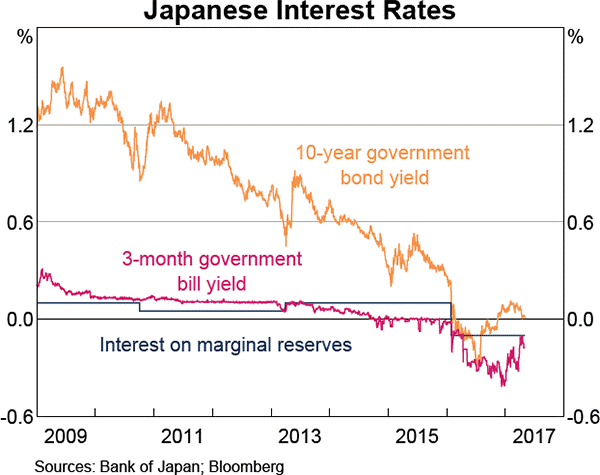# Fight Finance

#### CoursesTagsRandomAllRecentScores

For a price of $102, Andrea will sell you a share which just paid a dividend of$10 yesterday, and is expected to pay dividends every year forever, growing at a rate of 5% pa.

So the next dividend will be $10(1+0.05)^1=10.50$ in one year from now, and the year after it will be $10(1+0.05)^2=11.025$ and so on.

The required return of the stock is 15% pa.

Would you like to the share or politely ?

A share just paid its semi-annual dividend of $10. The dividend is expected to grow at 2% every 6 months forever. This 2% growth rate is an effective 6 month rate. Therefore the next dividend will be$10.20 in six months. The required return of the stock 10% pa, given as an effective annual rate.

What is the price of the share now?

A share was bought for $10 (at t=0) and paid its annual dividend of$0.50 one year later (at t=1). Just after the dividend was paid, the share price was $11 (at t=1). What was the total return, capital return and income return? Calculate your answers as effective annual rates. The choices are given in the same order: $r_\text{total}$, $r_\text{capital}$, $r_\text{dividend}$. Which one of the following bonds is trading at a discount? Examine the following graph which shows stocks' betas $(\beta)$ and expected returns $(\mu)$:Assume that the CAPM holds and that future expectations of stocks' returns and betas are correctly measured. Which statement is NOT correct? Which of the following statements is NOT equivalent to the yield on debt? Assume that the debt being referred to is fairly priced, but do not assume that it's priced at par. Which of the following is NOT a synonym of 'required return'? You deposit cash into your bank account. Have you or debt? Radio-Rentals.com offers the Apple iphone 5S smart phone for rent at$12.95 per week paid in advance on a 2 year contract. After renting the phone, you must return it to Radio-Rentals.

Kogan.com offers the Apple iphone 5S smart phone for sale at \$699. You estimate that the phone will last for 3 years before it will break and be worthless.

Currently, the effective annual interest rate is 11.351%, the effective monthly interest rate 0.9% and the effective weekly interest rate is 0.207%. Assume that there are exactly 52 weeks per year and 12 months per year.

Find the equivalent annual cost of renting the phone and also buying the phone. The answers below are listed in the same order.

Refer to the below graph when answering the questions.Which of the following statements is NOT correct?

Copyright © 2014 Keith Woodward